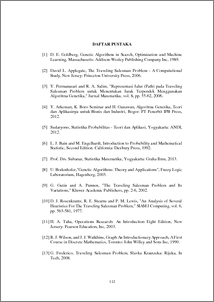# ANALYSIS OF HOW THE GENETIC ALGORITHM WORKS AND ITS APPLICATION FOR THE TRAVELING SALESMAN PROBLEM (TSP)

Nissa, Nuzulul Khairu (2019) ANALYSIS OF HOW THE GENETIC ALGORITHM WORKS AND ITS APPLICATION FOR THE TRAVELING SALESMAN PROBLEM (TSP). Undergraduate thesis, UNDIP.Preview
PDF
199KbPDF
Restricted to Repository staff only

2100KbPreview
PDF
113Kb

## Abstract

Genetic algorithm is a metaheuristic search algorithm based on the mechanism of natural selection and genetic operations to get a solution. The solutions obtained from genetic algorithms are determined by the operator and the parameters used. Determination of the workings of each operator, namely selection, crossover and mutation, can be known by using the Schemata theory. In addition, if it is assumed that the crossover process is ignored with the intention of being emphasized in the mutation process, the probability of the solution being produced will increase. Furthermore, if it is assumed that there is an Elitism process, the expected value is obtained that the genetic algorithm will converge at an optimal value. The example problem that can be solved using genetic algorithms is the Traveling Salesman Problem (TSP). TSP is an optimization problem, in order to find Hamilton cycle which has a minimum weight on a connected graph. The purpose of TSP is to find the shortest route from the city of origin to another city that will be addressed by a salesman provided that each city is only visited once and must return to the city of origin. The conventional method that will be used as a comparison of calculations to solve TSP problems is the Nearest Neighbor method. However, if the number of cities gets bigger, the use of the method becomes less efficient because more and more possible permutation routes are generated. From the calculation results, it was found that TSP resolution using genetic algorithms yielded a good and efficient solution when compared to the Nearest Neighbor method. Keywords: Genetic Algorithm, Traveling Salesman Problem (TSP), Nearest Neighbor

Item Type: Thesis (Undergraduate) Q Science > QA Mathematics Faculty of Science and Mathematics > Department of Mathematics 84269 INVALID USER 13 Jun 2022 11:14 13 Jun 2022 11:14

Repository Staff Only: item control page